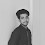# URI / BEE CROWD 1101 - Sequence of Numbers and Sum - Solution in C,C++,Python | URI - BEECROWD - BEE 1101 Solution in C,C++,Python

beecrowd | 1101

# Adapted by Neilor Tonin, URI  Brazil

Timelimit: 1

URI / BEE CROWD 1101 - Sequence of Numbers and Sum - Solution in C,C++,Python | URI - BEECROWD - BEE 1099 Solution in C,C++,Python:

Read an undetermined number of pairs values and N (stop when any of these values is less or equal to zero). For each pair, print the sequence from the smallest to the biggest (including both) and the sum of consecutive integers between them (including both).

## Input

The input file contains pairs of integer values and N. The last line of the file contains a number zero or negative, or both.

## Output

For each pair of numbers, print the sequence from the smallest to the biggest and the sum of these values, as shown below.

 Input Sample Output Sample 5 26 35 0 2 3 4 5 Sum=143 4 5 6 Sum=18

Demonstration:

This program takes user inputs . Then check the conditions for each test case contains two integer number x and y and compare those two numbers if x is greater than y , then swapping in ascending order and calculating the sum of all  numbers .
Finally print the result.

N.B: Don't copy paste the code as same. Just try to understand it and try yourself.

## URI / BEECROWD Online Judge 1101 Solve in C :

```#include <stdio.h>

int main(){

int n;
int x, y, aux;
int soma;

while(1){

scanf("%d%d",&x,&y);
if(x <= 0) break;
if(y <= 0) break;

if(x > y){
aux = x;
x = y;
y = aux;
}
soma = 0;
for(int i = x; i <= y; i++){
printf("%d ",i);
soma += i;
}
printf("Sum=%d\n",soma);

}
return 0;
}```

### 2 Responses to URI / BEE CROWD 1101 - Sequence of Numbers and Sum - Solution in C,C++,Python

1.What aux means here?

2.aux is a temporary variable for swapping the values.